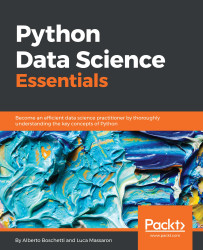•#### Python Data Science Essentials#### Overview of this book

The book starts by introducing you to setting up your essential data science toolbox. Then it will guide you across all the data munging and preprocessing phases. This will be done in a manner that explains all the core data science activities related to loading data, transforming and fixing it for analysis, as well as exploring and processing it. Finally, it will complete the overview by presenting you with the main machine learning algorithms, the graph analysis technicalities, and all the visualization instruments that can make your life easier in presenting your results. In this walkthrough, structured as a data science project, you will always be accompanied by clear code and simplified examples to help you understand the underlying mechanics and real-world datasets.
Python Data Science EssentialsCreditswww.PacktPub.comPrefaceFree Chapter
First StepsData MungingThe Data Science PipelineMachine LearningSocial Network AnalysisVisualizationIndex## NumPy fast operation and computations

When arrays need to be manipulated by mathematical operations, you just need to apply the operation on the array with respect to a numerical constant (a scalar) or an array of the exact same shape:

```In: import numpy as np
In: a =  np.arange(5).reshape(1,5)
In: a += 1
In: a*a
Out: array([[ 1,  4,  9, 16, 25]])
```

The result will be that the operation will be performed element-wise, that is, every element of the array is operated by either the scalar value or the corresponding element of the other array.

When operating on arrays of different dimensions, it is still possible to obtain element-wise operations without having to restructure the data in case one of the corresponding dimensions is 1. In fact, in such a case, the dimension of size 1 is stretched until it matches the dimension of the corresponding array. This conversion is called broadcasting.

For instance:

```In: a = np.arange(5).reshape(1,5) + 1
b = np.arange(5).reshape(5,1) + 1
a * b
Out: array([[ 1...```